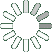Puzzle Command
Daily Puzzle
Interesting Equation
April 2, 2015It looks like someone has been writing nonsense equations in a school for the gifted children. In certain circumstances these equations are actually correct.

10 - 6 = 4 10 + 6 = 4

11 + 6 = ?

Puzzle Notes
The equations make sense in certain circumstanced.
Think beyond the simple numbers to find your answer.
Enter in your solution below and click submit.